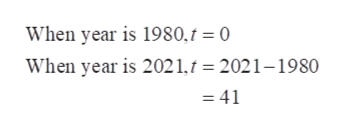# In 1980, the population of blue whales in the southern hemisphere was thought to number 4500. The population N(t) has been decreasing according to the formulaN(t) = 4500e−0.1345t,where t is in years and t = 0 corresponds to 1980. Predict the population in the year 2021 if this trend continues. (Round your answer to the nearest whole number.)

Question
8 views

In 1980, the population of blue whales in the southern hemisphere was thought to number 4500. The population N(t) has been decreasing according to the formula

N(t) = 4500e−0.1345t,

where t is in years and t = 0 corresponds to 1980. Predict the population in the year 2021 if this trend continues. (Round your answer to the nearest whole number.)

check_circle

Step 1

First of all, find the value of t for...help_outlineImage TranscriptioncloseWhen year is 1980,t = 0 When year is 2021,t = 2021–1980 = 41 fullscreen

### Want to see the full answer?

See Solution

#### Want to see this answer and more?

Solutions are written by subject experts who are available 24/7. Questions are typically answered within 1 hour.*

See Solution
*Response times may vary by subject and question.
Tagged in

### Other